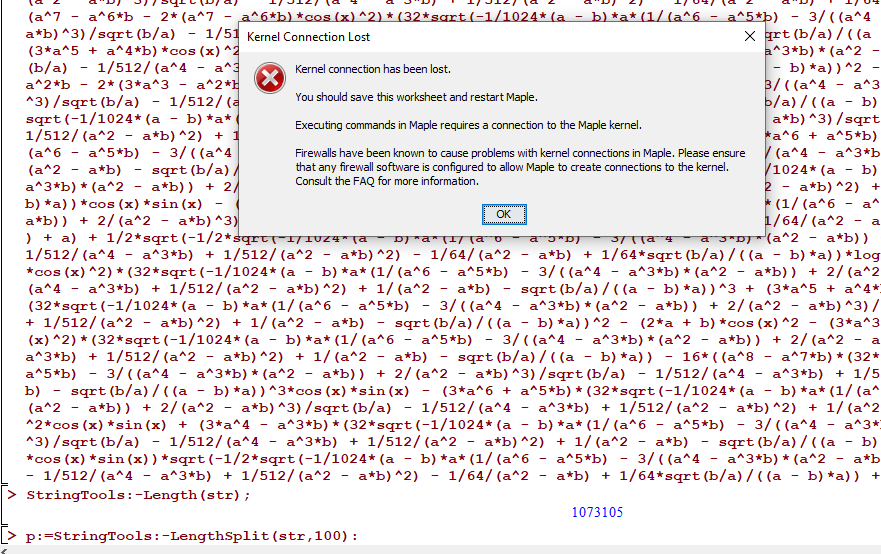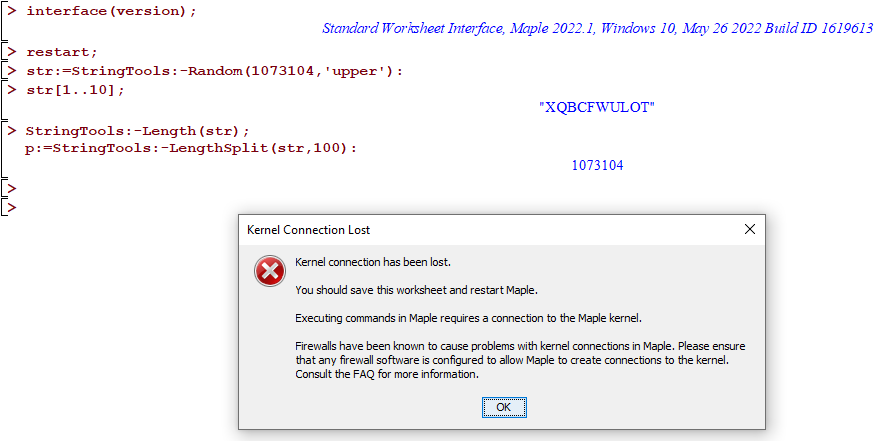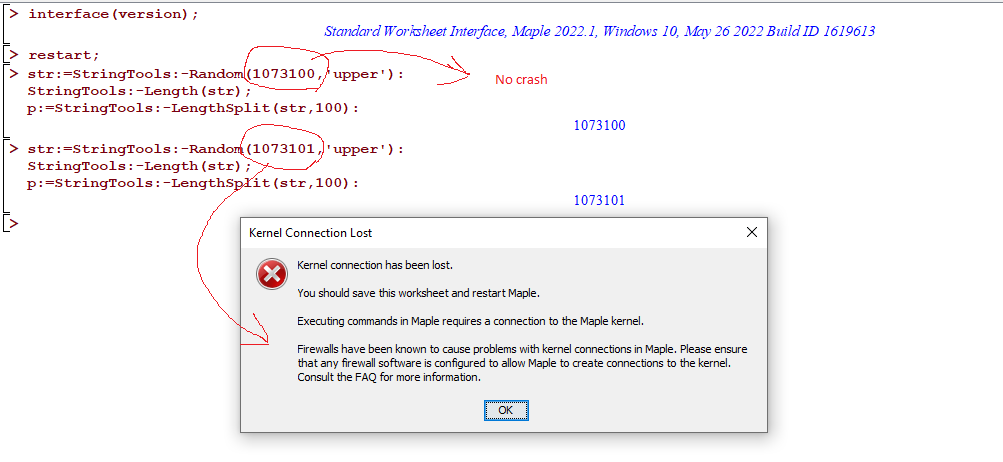## system of equations...

Dear all

How can I solve the system of equations with unknowns  c and Z

system_eqs.mw

Maybe the question can be solved by hand.

thanks

## fsolve is not working...

Hello Everyone;

Hope you are fine. I need to solve the system of equation. I am using fsolve command but it is not working. Kindly guide me.

Thanks

ques.mw

 >>>>>>>>>>(1)
 >>## Is it possible to fit data with units?...

Fitting procedures in Maple are self explaning and easy to perform. However, I was wondering if it is possible to fit data vectors with units. For example one vector would have units of seconds and the other units of length. Thank you for your help.

## Extract domain number...

Is there any command that allows me to extract the input number of any function f?

exmple:

f: (x1; x2;...;xn)-> y

command (f)=n

thanks for the help!

## Why Maple crash when doing String Split on string ...

I have string of length

StringTools:-Length(str);
1073105

When doing

p:=StringTools:-LengthSplit(str,100):

Maple givesFrom help:

LengthSplit
split a string into equal length pieces

This is all done in .mpl  file actually, not interactive, but I made the above worksheet to show  the problem. The string is read from a file by a program and it wants to split it to 100 length smaller strings.

I have lots of RAM. 128 GB.  So why does Maple crash and is there a workaround this?

This string was generated by another software.

I have attached the above example below as worksheet.  Maple 2022.1 on windows 10.

Here is a simpler way to generate this problem

```restart;
str:=StringTools:-Random(1073104,'upper'):
str[1..10];

StringTools:-Length(str);
p:=StringTools:-LengthSplit(str,100):
```Using trial and error, the crash happens when the length reaches 1073101. At 1073100 there was no crash.## Binary Recursive algorithm ...

I need to define a simple recursive algorithm (i'm not a programmer) such that:

xi=xi-1+b

with i=0,1,...,n;

and with all xi's elements of a Set A

how can i achieve this?

## Could numer and denom be made to work with inerts?...

I have some expressions like 3%/5 and would like to get the numerator and denominators numer(3%/5) does not return 3.

I cannot evaluate it since it might reduce the fraction which will make my results invalid.

## different coeff...

Hi,

I want to get the coefficient of some terms but I can't define suitable codes to do it:

I used the codes of one question on this site namely

https://www.mapleprimes.com/questions/229770-How-To-Find-The-Coefficient-Of-X-X2and

but they do not work for my case.

My case is as follows:

restart;
F:=2*y*sin(beta*x)+6*z*cos(beta*x)+24*sin(beta*x)*cos(beta*x):

coeff(F,sin(beta*x));
2 y + 24 cos(beta x)
coeff(F,cos(beta*x));
6 z + 24 sin(beta x)

coeff(coeff(F,cos(beta*x)),sin(beta*x));
24
The last one is true but for the first two cases, I want to get 2y and 6z respectively.